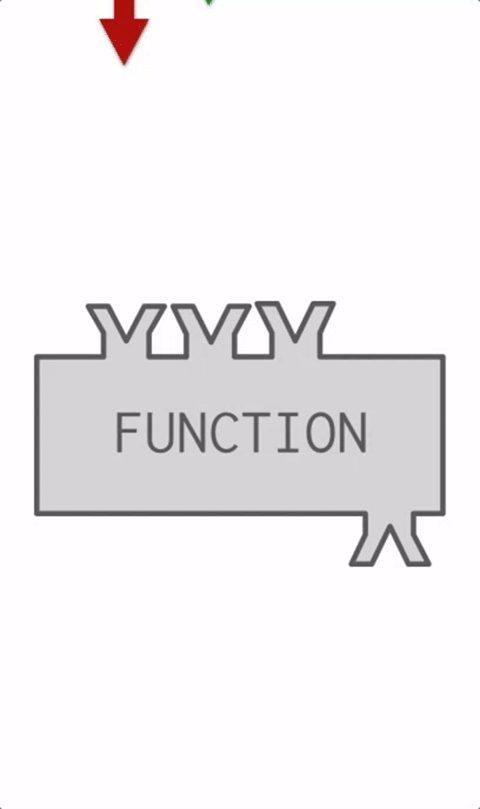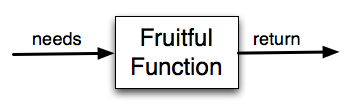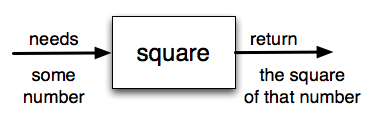# 12.5. Returning a value from a function¶Not only can you pass a parameter value into a function, a function can also produce a value. You have already seen this in some previous functions that you have used. For example, len takes a list or string as a parameter value and returns a number, the length of that list or string. range takes an integer as a parameter value and returns a list containing all the numbers from 0 up to that parameter value.

Functions that return values are sometimes called fruitful functions. In many other languages, a function that doesn’t return a value is called a procedure, but we will stick here with the Python way of also calling it a function, or if we want to stress it, a non-fruitful function.How do we write our own fruitful function? Let’s start by creating a very simple mathematical function that we will call square. The square function will take one number as a parameter and return the result of squaring that number. Here is the black-box diagram with the Python code following.The return statement is followed by an expression which is evaluated. Its result is returned to the caller as the “fruit” of calling this function. Because the return statement can contain any Python expression we could have avoided creating the temporary variable y and simply used return x*x. Try modifying the square function above to see that this works just the same. On the other hand, using temporary variables like y in the program above makes debugging easier. These temporary variables are referred to as local variables.

Notice something important here. The name of the variable we pass as an argument — toSquare — has nothing to do with the name of the formal parameter — x. It is as if x = toSquare is executed when square is called. It doesn’t matter what the value was named in the caller (the place where the function was invoked). Inside square, it’s name is x. You can see this very clearly in codelens, where the global variables and the local variables for the square function are in separate boxes.

Activity: CodeLens 12.5.3 (clens11_4_1)

There is one more aspect of function return values that should be noted. All Python functions return the special value None unless there is an explicit return statement with a value other than None. Consider the following common mistake made by beginning Python programmers. As you step through this example, pay very close attention to the return value in the local variables listing. Then look at what is printed when the function is over.

Activity: CodeLens 12.5.4 (clens11_4_2)

The problem with this function is that even though it prints the value of the squared input, that value will not be returned to the place where the call was done. Instead, the value None will be returned. Since line 6 uses the return value as the right hand side of an assignment statement, squareResult will have None as its value and the result printed in line 7 is incorrect. Typically, functions will return values that can be printed or processed in some other way by the caller.

A return statement, once executed, immediately terminates execution of a function, even if it is not the last statement in the function. In the following code, when line 3 executes, the value 5 is returned and assigned to the variable x, then printed. Lines 4 and 5 never execute. Run the following code and try making some modifications of it to make sure you understand why “there” and 10 never print out.

The fact that a return statement immediately ends execution of the code block inside a function is important to understand for writing complex programs, and it can also be very useful. The following example is a situation where you can use this to your advantage – and understanding this will help you understand other people’s code better, and be able to walk through code more confidently.

Consider a situation where you want to write a function to find out, from a class attendance list, whether anyone’s first name is longer than five letters, called longer_than_five. If there is anyone in class whose first name is longer than 5 letters, the function should return True. Otherwise, it should return False.

In this case, you’ll be using conditional statements in the code that exists in the function body, the code block indented underneath the function definition statement (just like the code that starts with the line print("here") in the example above – that’s the body of the function weird, above).

Bonus challenge for studying: After you look at the explanation below, stop looking at the code – just the description of the function above it, and try to write the code yourself! Then test it on different lists and make sure that it works. But read the explanation first, so you can be sure you have a solid grasp on these function mechanics.

First, an English plan for this new function to define called longer_than_five:

• You’ll want to pass in a list of strings (representing people’s first names) to the function.

• You’ll want to iterate over all the items in the list, each of the strings.

• As soon as you get to one name that is longer than five letters, you know the function should return True – yes, there is at least one name longer than five letters!

• And if you go through the whole list and there was no name longer than five letters, then the function should return False.

Now, the code:

So far, we have just seen return values being assigned to variables. For example, we had the line squareResult = square(toSquare). As with all assignment statements, the right hand side is executed first. It invokes the square function, passing in a parameter value 10 (the current value of toSquare). That returns a value 100, which completes the evaluation of the right-hand side of the assignment. 100 is then assigned to the variable squareResult. In this case, the function invocation was the entire expression that was evaluated.

Function invocations, however, can also be used as part of more complicated expressions. For example, squareResult = 2 * square(toSquare). In this case, the value 100 is returned and is then multiplied by 2 to produce the value 200. When python evaluates an expression like x * 3, it substitutes the current value of x into the expression and then does the multiplication. When python evaluates an expression like 2 * square(toSquare), it substitutes the return value 100 for entire function invocation and then does the multiplication.

To reiterate, when executing a line of code squareResult = 2 * square(toSquare), the python interpreter does these steps:

1. It’s an assignment statement, so evaluate the right-hand side expression 2 * square(toSquare).

2. Look up the values of the variables square and toSquare: square is a function object and toSquare is 10

3. Pass 10 as a parameter value to the function, get back the return value 100

4. Substitute 100 for square(toSquare), so that the expression now reads 2 * 100

5. Assign 200 to variable squareResult

8. Write a function named same that takes a string as input, and simply returns that string.

9. Write a function called same_thing that returns the parameter, unchanged.

10. Write a function called subtract_three that takes an integer or any number as input, and returns that number minus three.

11. Write a function called change that takes one number as its input and returns that number, plus 7.

12. Write a function named intro that takes a string as input. This string ist intended to be a person’s name and the output is a standardized greeting. For example, given the string “Becky” as input, the function should return: “Hello, my name is Becky and I love SI 106.”

13. Write a function called s_change that takes one string as input and returns that string, concatenated with the string “ for fun.”.

14. Write a function called decision that takes a string as input, and then checks the number of characters. If it has over 17 characters, return “This is a long string”, if it is shorter or has 17 characters, return “This is a short string”.